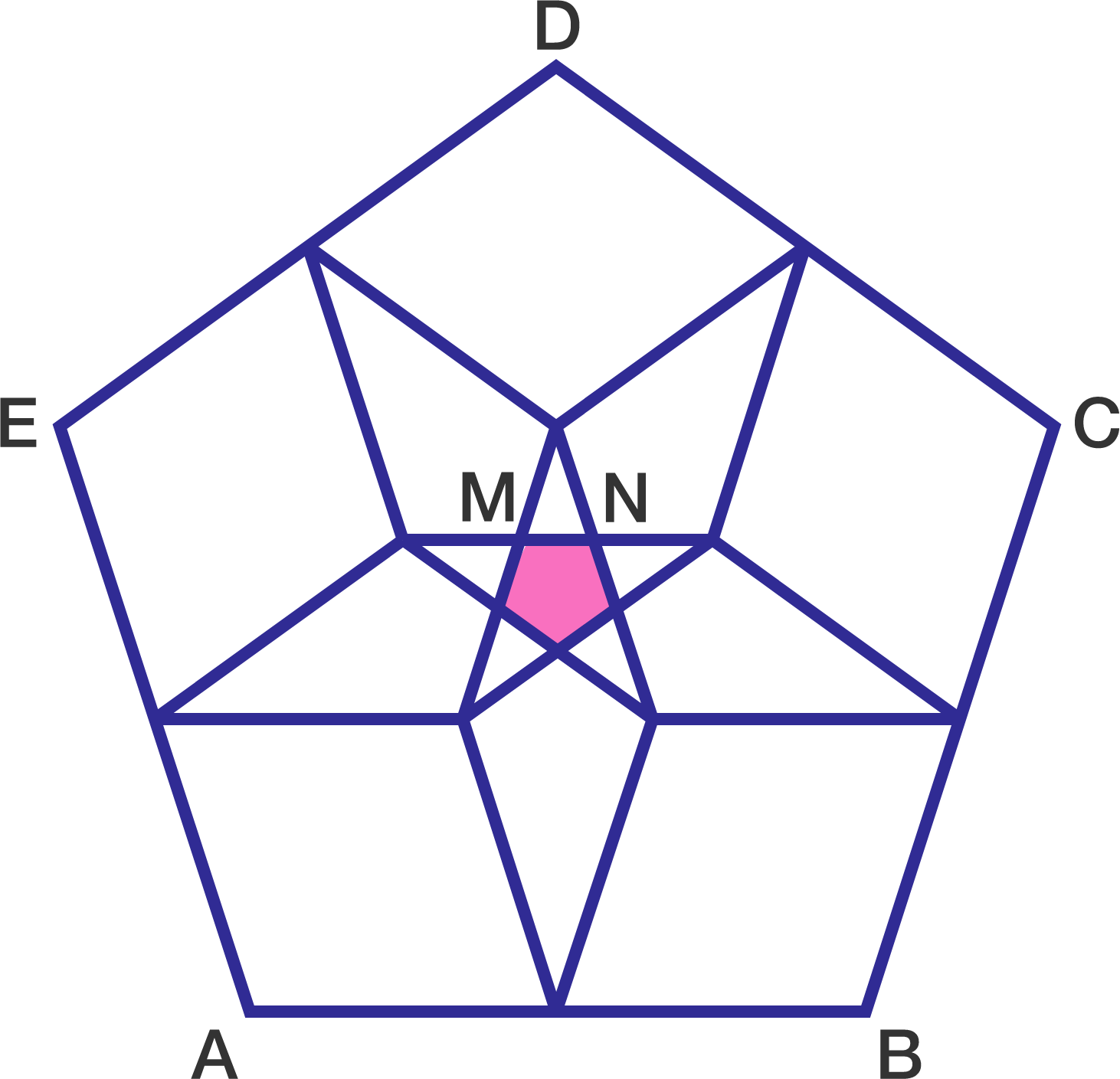# Pentagons Inside Pentagon

Geometry Level 3Inside a regular pentagon $ABCDE$, construct 5 more regular pentagons of side length $\dfrac{1}{2}AB$. The part of the overlapping of these pentagons yields another regular pentagon of side length $MN$.

Let $r=\dfrac{AB}{MN}$. Find $\displaystyle \left \lfloor 1000r \right \rfloor$.

×